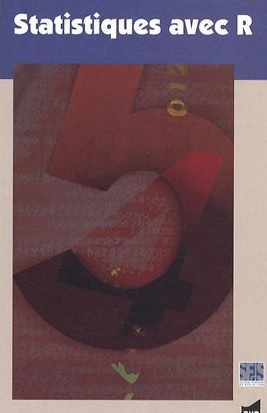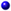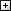Processing ......FreeComputerBooks.com Free Computer, Mathematics, Technical Books and Lecture Notes, etc.

Statistics with R
For Weather, Flights, Runways of Airports all over the world, etc, click here.
• Title Statistics with R
• Author(s) Vincent Zoonekynd, Jos� Gama
• Publisher: Vincent Zoonekynd and �bo Akademi University
• Hardcover/Paperback N/A
• eBook HTML and PDF
• Language: English
• ISBN-10: N/A
• ISBN-13: N/ABook Description

This book provides an elementary-level introduction to R, targeting both non-statistician scientists in various fields and students of statistics.

The main mode of presentation is via code examples with liberal commenting of the code and the output, from the computational as well as the statistical viewpoint. Brief sections introduce the statistical methods before they are used. A supplementary R package can be downloaded and contains the data sets.

All examples are directly runnable and all graphics in the text are generated from the examples. The statistical methodology covered includes statistical standard distributions, one- and two-sample tests with continuous data, regression analysis, one-and two-way analysis of variance, regression analysis, analysis of tabular data, and sample size calculations. In addition, the last four chapters contain introductions to multiple linear regression analysis, linear models in general, logistic regression, and survival analysis.All CategoriesRecent BooksIT Research LibraryMiscellaneous BooksComputer LanguagesComputer ScienceData Science/DatabasesElectronic EngineeringJava and Java EE (J2EE)Linux and UnixMathematicsMicrosoft and .NETMobile ComputingNetworking and CommunicationsSoftware EngineeringSpecial TopicsWeb Programming